# Geometric and Algebraic Meaning of Determinants

A recent question led me to look back in the Ask Dr. Math archives for questions about the definition and deeper meaning of determinants. Next week, we’ll see another old question for additional background, followed by the new question.

## What is a determinant? Two perspectives

First, a question from 1997:

Determinant of a Matrix

My Algebra 2 teacher told us that for extra credit we could give him a complete and unabridged version of the definition of a DETERMINANT of a matrix. He said that we could only find it in a library or an advanced collegiate math book. He also said that if we could understand the definition, it wasn't the right one.

I have tried numerous dictionaries and other references over the Internet. Please help me if you can! Thank you so much.

Can we give a definition that can’t be understood by a student at this introductory level, and also make it understandable? We’ll see.

### Geometric definition: Length, area, volume

Hi Mark,

I'll give you two definitions that are exactly equivalent, but sound very different.

The first is geometric. I assume you've plotted things in an x-y coordinate system, right? I assume you can imagine doing the same thing in three dimensions with an x-y-z coordinate system as well.

In 2-D, when you talk about the point (2, 4), you can think of the "2" and "4" as directions to get from the origin to the point - "move 2 units in the x direction and 4 in the y direction."  In a 3-D system, the same idea holds - (1, 3, 7) means start at the origin (0,0,0), go 1 unit in the x direction, 3 in the y direction, and 7 in the z direction.

Similarly, you could have coordinates in one dimension, but there's just one number.

We’ll be looking at one-, two-, and three-dimensional “parallelotopes“, which are line segments, parallelograms, and parallelepipeds, respectively.

The determinant of a 1x1 matrix is the signed length of the line from the origin to the point. It's positive if the point is in the positive x direction, negative if in the other direction.

Here is a one-dimensional parallelotope, a segment, defined by a single point, or vector (in this case, a negative number), which can be represented as a $$1\times1$$ matrix $$\begin{bmatrix}x_1\end{bmatrix}$$: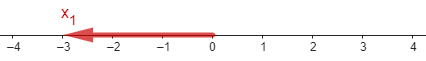The determinant $$\det\left(\begin{bmatrix}-3\end{bmatrix}\right)=\begin{vmatrix}-3\end{vmatrix}$$ (not to be confused with an absolute value!) is $$-3$$, the signed length of the segment.

In 2-D, look at the matrix as two 2-dimensional points on the plane, and complete the parallelogram that includes those two points and the origin. The (signed) area of this parallelogram is the determinant.  If you sweep clockwise from the first to the second, the determinant is negative; otherwise, positive.

Here is a two-dimensional parallelotope, a parallelogram, defined by two vectors (pairs of numbers), which can be represented by a $$2\times2$$ matrix, $$\displaystyle\begin{bmatrix}x_1&y_1\\x_2&y_2\end{bmatrix}$$: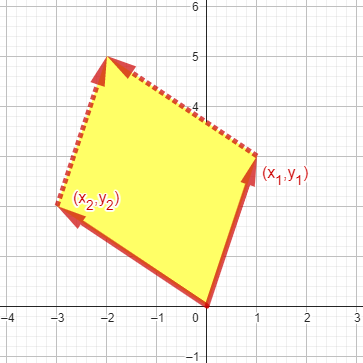The determinant $$\displaystyle\begin{vmatrix}1&3\\-3&2\end{vmatrix}$$ is $$11$$, its area. If we reverse the order, to $$\displaystyle\begin{vmatrix}-3&2\\1&3\end{vmatrix}$$, we get $$-11$$, because we would be going around the figure clockwise rather than counterclockwise as shown.

In 3-D, look at the matrix as 3 3-dimensional points in space.  Complete the parallelepiped that includes these points and the origin, and the determinant is the (signed) volume of the parallelepiped.

Here is a three-dimensional parallelotope, a parallelepiped, defined by three vectors (triples of numbers), which can be represented by a $$3\times3$$ matrix, $$\displaystyle\begin{bmatrix}x_1&y_1&z_1\\x_2&y_2&z_2\\x_3&y_3&z_3\end{bmatrix}$$: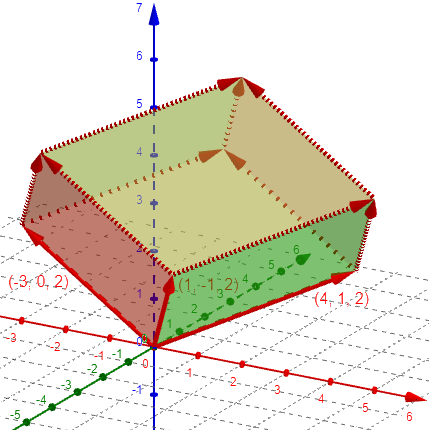The determinant $$\displaystyle\begin{vmatrix}-3&0&2\\1&-1&2\\4&1&2\end{vmatrix}$$ is $$22$$, its signed volume. (Just trust me on that number.)

The same idea works in any number of dimensions.  The determinant is just the (signed) volume of the n-dimensional parallelepiped.

Notice that length, area, volume are the "volumes" in 1-, 2-, and 3-dimensional spaces.  A similar concept of volume exists for Euclidean space of any dimensionality.

He’s only defined the determinant as the volume, without showing how to actually calculate it. The second definition will allow us to do so, and next week we’ll see why.

### Algebraic definition: Sum of products

Doctor Tom continued:

Okay. That's the geometric definition. I like it because I can make a mental picture of it. Here's the algebraic definition:

I'll do it in 3 dimensions, but exactly the same idea works in any number of dimensions.  Let's look at the determinant of this matrix:

| a11 a12 a13 |
| a21 a22 a23 |
| a31 a32 a33 |

The numbers after the "a" are the row and column numbers.

These are normally written as subscripts: $$\begin{vmatrix}a_{11}&a_{12}&a_{13}\\a_{21}&a_{22}&a_{23}\\a_{31}&a_{32}&a_{33}\end{vmatrix}$$

A permutation of a set of numbers is a re-arrangement.

For example, there are 6 permutations of the list (1 2 3), including the "re-arrangement" that leaves everything unchanged). Ignore for the moment the "+1" and "-1" after each one:

(1 2 3) -> (1 2 3)   +1
(1 2 3) -> (1 3 2)   -1
(1 2 3) -> (2 1 3)   -1
(1 2 3) -> (2 3 1)   +1
(1 2 3) -> (3 1 2)   +1
(1 2 3) -> (3 2 1)   -1

Now imagine that you start with three objects labelled 1, 2, and 3 arranged as they are on the left, and need to convert them to the order on the right, but you're only allowed to swap one pair at a time. To get to the final arrangement, you'll find that there are lots of ways to do it, but every way (for a particular rearrangement) always requires an even number of swaps or always requires an odd number of swaps. I've labelled those that always need an even number of swaps with +1 and those needing an odd number as -1 above.

For example, to change (1 2 3) to (3 1 2), you might swap 2 and 3 to get (1 3 2), then swap 1 and 3 to get (3 1 2). This is an even number of swaps, so it is an even permutation, and is labeled with $$+1$$. To get from there to (3 2 1) would require a third swap, making it odd.

Now write down 6 products of the "a" terms, where the first number for each term is 1, 2, 3 and the second number is the rearrangement above for each of the six rearrangements.

Here's what they are, in the same order as above.  Be sure you understand this step:

a11*a22*a33
a11*a23*a32
a12*a21*a33
a12*a23*a31
a13*a21*a32
a13*a22*a31

The next to last row, for example, represents our (3 1 2), because the first indices are 1, 2, 3, while the second indices are 3, 1, 2.

The determinant is just the sum of all 6 terms, but put a "+" in front if the rearrangement is even, and a "-" in front if the rearrangement required an odd number of swaps.

+a11*a22*a33 -a11*a23*a32
-a12*a21*a33 +a12*a23*a31
+a13*a21*a32 -a13*a22*a31

You could say that we are adding all the products of even permutations, and subtracting the products of odd permutations.

Here is how we evaluate the determinant for volume in the example above:

$$\displaystyle\begin{vmatrix}-3&0&2\\1&-1&2\\4&1&2\end{vmatrix}=$$ $$(-3)(-1)(2)-(-3)(2)(1)\\-(0)(1)(2)+(0)(2)(4)\\+(2)(1)(1)-(2)(-1)(4)=$$ $$6+6-0+0+2+8=22$$

For a 4x4 matrix, there will be 24 rearrangements, like this:

(1 2 3 4) -> (3 2 4 1) +1
...

so there will be 24 terms in the expression of the determinant.

For a 5x5 matrix there are 120 rearrangements, so there will be 120 terms in the determinant, and so on.

For an NxN matrix, there will be N! (N factorial) terms, where factorial means you multiply together all the terms from N down to 1.  For example, 5! = "5 factorial" = 5x4x3x2x1 = 120.

Now, Doctor Tom has declared that these two definitions are equivalent, but not proved it; that fact is demonstrated, for two dimensions, in Polygon Coordinates and Areas, where we derive various formulas, one of which is our determinant. We’ll see a three-dimensional explanation later. For now, we are just trusting that he is right.

## Geometric definition, algebraic properties

Two weeks later, we got a similar question, and Doctor Tom gave a deeper answer, because this was a teacher rather than a student:

Explaining the Determinant

I am trying to understand what the determinant of a matrix actually is. I have a degree in mathematics and am currently teaching Algebra II to gifted and talented students. I know how to find the determinant and how to teach the process of finding the determinant, but I haven't been able  to explain what it is. Please help.

Interestingly, I found in a History of Math text, From Five Fingers to Infinity, that before Arthur Cayley "created" matrix theory, Leibniz and a Chinese or Japanese mathematician simultaneously and independently discovered the determinant. How could the determinant have been discovered before the matrix? I guess I have two questions.

What is it, and how was it discovered without matrices, when the determinant is a property of a matrix? Two good questions!

Doctor Tom answered, starting with the geometric definition we saw above:

Hello Jeremy,

I always think of it geometrically.

Let's look in two dimensions, at the determinant of the following:

| x0 y0 | = x0*y1 - x1*y0
| x1 y1 |

Now imagine the two vectors (x0, y0) and (x1, y1) drawn in the x-y plane from the origin. If you consider them to be two sides of a parallelogram, then the determinant is the area of the parallelogram.  Well, not exactly the area, the "signed" area, in the sense that if you sweep the area clockwise, you get one sign, and the opposite sign if you sweep it in the other direction. It's just as useful a concept as considering area below the x-axis as negative in your calculus course.

Swapping the vectors swaps the sign, in the same way that swapping the rows of the determinant swaps the sign.

This is an algebraic property of determinants; so the two perspectives are compatible at least in this.

In one dimension, the determinant is just the number, but if you "plot" that number on a number line, it's the (signed) length of the line. If it goes in the positive direction from the origin, it's positive, and negative otherwise.

In three dimensions, consider three vectors (x0,y0,z0), (x1,y1,z1), and (x2,y2,z2). If you draw them from the origin, they form the principal edges of a parallelepiped, and the determinant of:

| x0 y0 z0 |
| x1 y1 z1 |
| x2 y2 z2 |

is the volume of that parallelepiped.

In higher dimensions, its just the 4D (or 5D, or 6D ...) signed "hypervolumes" of the hyper-parallelepipeds.

It isn’t quite so clear how a positive or negative signed volume can be identified, but they can.

Taking that as the definition, we can derive algebraic properties, one of which we already saw for two dimensions.

With this view, it's easy to see why the determinant's properties make sense. Swapping two rows changes the order of sweeping out the volume, and will hence turn a positive volume to negative or vice-versa.

For example, here is the value of the determinant above, with the first two rows swapped:

$$\displaystyle\begin{vmatrix}1&-1&2\\-3&0&2\\4&1&2\end{vmatrix}=$$ $$(1)(0)(2)-(1)(2)(1)\\-(-1)(-3)(2)+(-1)(2)(4)\\+(2)(-3)(1)-(2)(0)(4)=$$ $$0-2-6-8-6-0=-22$$

It’s the negative of the original volume.

Multiplying all the elements of a row by a constant (say 2) stretches the parallelepiped by a factor of 2 in one direction, and hence doubles the volume.

Here I’ve doubled the length of the second row (vector) in the original determinant, which doubles the volume by stretching it in that direction: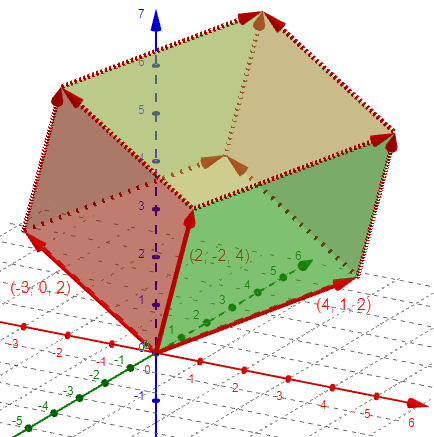$$\displaystyle\begin{vmatrix}-3&0&2\\2&-2&4\\4&1&2\end{vmatrix}=$$ $$(-3)(-2)(2)-(-3)(4)(1)\\-(0)(2)(2)+(0)(4)(4)\\+(2)(2)(1)-(2)(-2)(4)=$$ $$12+12-0+0+4+16=44$$

We can also multiply a column by a constant; here I’ve halved all z-coordinates from the determinant above, which halves the volume, back to what it was originally: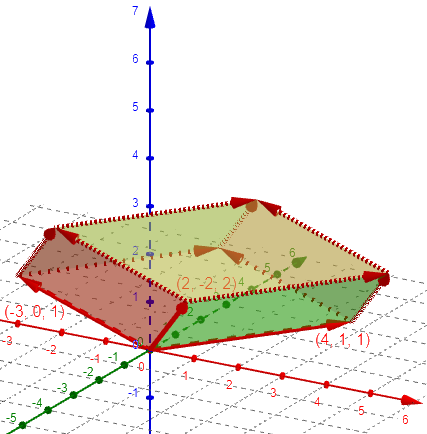$$\displaystyle\begin{vmatrix}-3&0&1\\2&-2&2\\4&1&1\end{vmatrix}=$$ $$(-3)(-2)(1)-(-3)(2)(1)\\-(0)(2)(1)+(0)(2)(4)\\+(1)(2)(1)-(1)(-2)(4)=$$ $$6+6-0+0+2+8=22$$

Adding a row to another just skews the parallelepiped parallel to one of its faces, and hence (Cavalieri's principle) leaves the volume unchanged. (If you can't see this, plot it in two dimensions for a couple of examples.)

Here I’ve added the second vector to the first in that last one: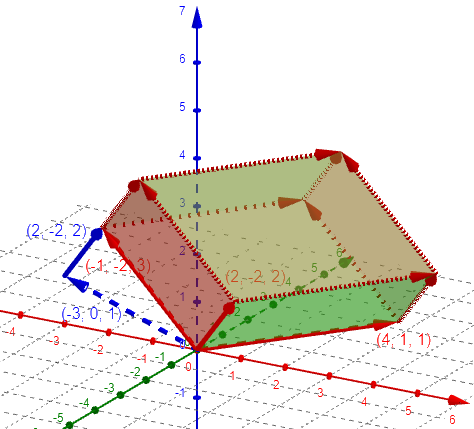Because it has been skewed parallel to two of its faces, cross-sections parallel to either face (say, the green ones) retain the same areas, and the volume is unchanged:

$$\displaystyle\begin{vmatrix}-1&-2&3\\2&-2&2\\4&1&1\end{vmatrix}=$$ $$(-1)(-2)(1)-(-1)(2)(1)\\-(-2)(2)(1)+(-2)(2)(4)\\+(3)(2)(1)-(3)(-2)(4)=$$ $$2+2+4-16+6+24=22$$

Cavalieri’s principle has been mentioned in Volume and Surface Area of a Sphere – Without Calculus. It is easily understood if you imagine our figure as a stack of cards, and slide them in such a way that they retain the same area and thickness, but are stacked at a different angle. Here is an example of a cross-section of our figure, which has been slid upward parallel to the front edge: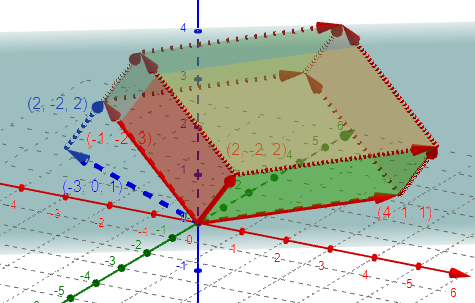I cut the figure with a light blue plane parallel to the green faces.

Check the other allowed determinant manipulations to see how they relate to the geometry.

Because a determinant is a fundamental geometric property of a collection of N N-dimensional vectors, it's not too surprising that different folks would stumble across it, even without knowing what a matrix is.

One place the determinant is used without formally thinking of matrices (specifically, as objects that can be added and multiplied) is in solving a system of equations using Cramer’s rule; the coefficients are already arranged in a rectangle, and their determinant arises naturally in the work of solving by elimination (along with the rules for manipulating them).

We’ve discussed in Polygon Coordinates and Areas how to use determinants to find the area of a triangle, and showed a couple proofs. We don’t have a proof that the determinant actually gives the volume of a parallelepiped as we normally think of it; but we see here that the algebraic properties of the determinant are in agreement with the geometric properties of the volume, supporting the equivalence.

Next week we’ll see a fuller explanation of that volume, before comparing areas and volumes.

### 1 thought on “Geometric and Algebraic Meaning of Determinants”

This site uses Akismet to reduce spam. Learn how your comment data is processed.